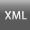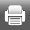Volume 14, Issue 2 (8-2020)2020, 14(2): 223-252 Back to browse issues page
Effect of Loading Waveform and Frequency on Dynamic Properties of Dry Sands Using Shaking Table Tests
Abstract:   (1697 Views)
Introduction
Material and methods
A hydraulic shaking table with a single degree of freedom, designed and constructed at the Crisis Management Center of Urmia University, was used to conduct the experiments. Firoozkuh No. 161 sand was used in all the experiments. The Firoozkuh sand gradation curve is similar to that of Toyoura sand. In this study, accelerometers were used to measure the acceleration of the input to the sample as well as to record the acceleration caused by the input excitation at different depths of the soil sample. The displacement transducers (LVDT sensors) were also used to measure linear displacement. Each soil sample was constructed using dry Firoozkuh sand poured uniformly into the container from four equal heights of 150 mm to reach a total height of 600 mm. During the compaction process, the accelerometers A1, A2, and A3 were placed at a depth of 150, 300 and 450 mm with respect to the bottom of container. Also, one accelerometer, A0, was attached rigidly to the container base to measure base acceleration. A displacement transducer (L1) was placed on the soil surface at a height of 600 mm from the floor of the container to measure the vertical displacement of the surface of the soil. In this study, 42 shaking table tests were performed to study the effect of loading frequency and waveform on dynamic properties of dry sand. The test samples were subjected to rectangular, sinusoidal and triangular loading at frequencies of 0.5 to 9 Hz and at input acceleration of 0.1g and 0.3 g.
Results and discussion
Given the importance of G-γ and D-γ curves in dynamic analyses, the changes in shear modulus with shear strain has been studied. The results show that the shear modulus increases as the frequency increases in all cases, and this increase is observed at lower frequencies and increases with increasing frequency. On the other hand, the shear modulus decreases with increasing shear strain. At a constant testing frequency, soil samples subjected to the rectangular waveform exhibited the largest shear modulus while the samples subjected to the triangular waveform showed the least shear modulus. The shear modulus of the samples under the sinusoidal waveform is barely more than the shear modulus of samples under triangular waveform. Moreover, by increasing the shear strain, the shear modulus values ​​of samples with different waveforms have become closer and the waveform effect is reduced. As for the effect of input acceleration on the shear modulus , increasing the input acceleration increases the shear strain and consequently, decreases the shear modulus in all states (the values ​​of shear modulus in various frequencies and the waveforms under the input acceleration of 0.1 g are larger than the shear modulus values ​​under the input acceleration of 0.3g). In the case of the damping ratio, the results show that, in all cases, damping ratio increases with shear strain. At low strain levels, the damping ratio values at various frequencies and waveforms are low and yet very close. At higher strain levels, the increase in frequency increases the damping ratio. This increase is more significant at higher frequencies. Also, the effect of waveform on the damping ratio is more apparent at larger shear strains, and at such shear strain levels, soil samples under rectangular loading exhibit the largest damping ratio. The damping ratio associated with the sinusoidal and triangular loading are also close to each other and it is a slightly larger for sinusoidal loading. On the other hand, the damping ratio increases with input acceleration. In addition, the effect of increased input acceleration on the increase in the damping ratio is more obvious at higher frequencies mainly due to the increase in shear strain.
Conclusion
In the present study, the effects of loading frequency and waveform on the dynamic properties of dry sand were investigated using shaking table tests. The following conclusions were drawn:
• The shear modulus increases with frequency. The trend is more obvious at larger frequencies. The effect of loading frequency on the damping ratio of the soil at low levels of strain is negligible, and at relatively large strain levels, damping ratio increases with loading frequency.
• Soil samples exhibit the highest shear modulus and damping ratio under rectangular loading. Therefore, in all the tested frequencies and input accelerations, the values of G and D for the rectangular waveforms are greater than those of the sinusoidal and triangular waveforms. The shear modulus and damping ratio for the sinusoidal waveforms are marginally greater than those of triangular waveforms, yet one can consider them practically similar.
• In all cases, the shear strain increased by increasing the amplitude of the input acceleration, and as a result, the shear modulus decreased and the damping ratio increased../files/site1/files/142/2.pdf
Keywords: Shaking table, dynamic properties, frequency, waveform, shear modulus, damping ratioRights and permissionsThis work is licensed under a Creative Commons Attribution-NonCommercial 4.0 International License.
 Rights and permissionsThis work is licensed under a Creative Commons Attribution-NonCommercial 4.0 International License.